# Osculating plane

(diff) ← Older revision | Latest revision (diff) | Newer revision → (diff)

at a pointof a curveThe plane having contact of orderwithat(see Osculation). The osculating plane can also be defined as the limit of a variable plane passing through three points ofas these points approach. Usually, a curve intersects the osculating plane at the point of contact (see Fig.).Figure: o070560a

Ifis given by equations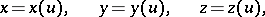then the equation of the osculating plane has the form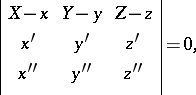where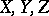are moving coordinates and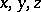,,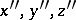are calculated at the point of contact. If all three coefficients ofin the equation of the osculating plane vanish, then the osculating plane becomes indefinite (and can coincide with any plane through the tangent line).